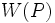# Strongly closed conjugacy functor

This article defines a property that can be evaluated for a conjugacy functor on a finite group. |View all such properties

## Definition

Suppose$G$ is a finite group,$p$ is a prime number, and$W$ is a conjugacy functor for the prime$p$. We say that$W$ is a strongly closed conjugacy functor if it satisfies the following equivalent conditions:

• There exists a$p$-Sylow subgroup$P$ of$G$ such that$W(P)$ is a strongly closed subgroup of$P$ relative to$G$.
• For every$p$-Sylow subgroup$P$ of$G$,$W(P)$ is a strongly closed subgroup of$P$ relative to$G$.

## Relation with other properties

### Stronger properties

Property Meaning Proof of implication Proof of strictness (reverse implication failure) Intermediate notions
conjugacy functor that gives a normal subgroup$W(P)$ is a normal subgroup of$G$. |FULL LIST, MORE INFO

### Weaker properties

Property Meaning Proof of implication Proof of strictness (reverse implication failure) Intermediate notions
weakly closed conjugacy functor$W(P)$ is weakly closed in$P$ relative to$G$. |FULL LIST, MORE INFO